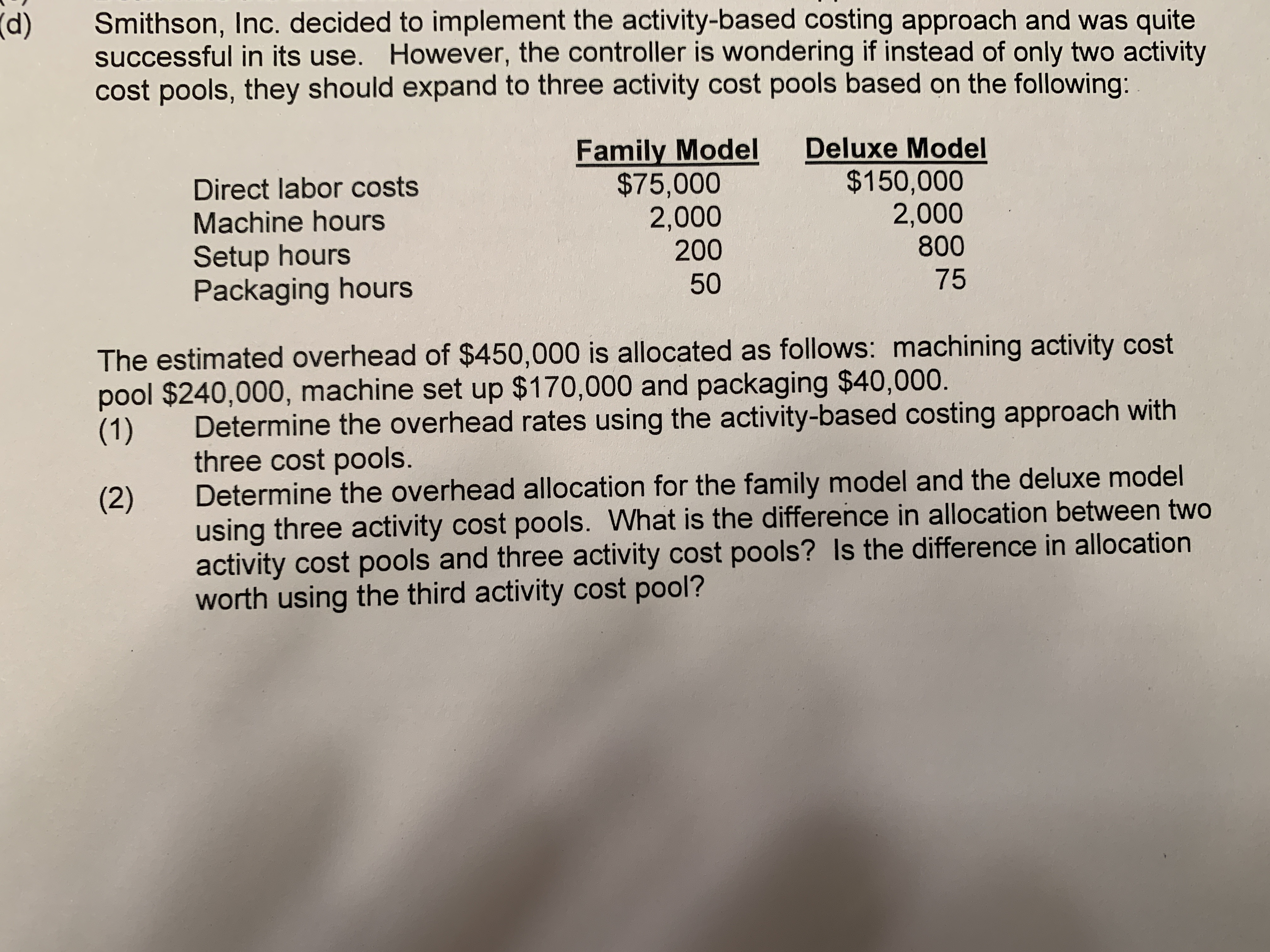# Smithson, Inc. decided to implement the activity-based costing approach and was quite successful in its use. However, the controller is wondering if instead of only two activity cost pools, they should expand to three activity cost pools based on the following: (d) Family Model $75,000 2,000 200 Deluxe Model$150,000 2,000 800 Direct labor costs Machine hours Setup hours Packaging hours 50 75 The estimated overhead of $450,000 is allocated as follows: machining activity cost pool$240,000, machine set up $170,000 and packaging$40,000. (1) Determine the overhead rates using the activity-based costing approach with three cost pools. Determine the overhead allocation for the family model and the deluxe model using three activity cost pools. What is the difference in allocation between two activity cost pools and three activity cost pools? Is the difference in allocation worth using the third activity cost pool? (2)

Questionhelp_outlineImage TranscriptioncloseSmithson, Inc. decided to implement the activity-based costing approach and was quite successful in its use. However, the controller is wondering if instead of only two activity cost pools, they should expand to three activity cost pools based on the following: (d) Family Model $75,000 2,000 200 Deluxe Model$150,000 2,000 800 Direct labor costs Machine hours Setup hours Packaging hours 50 75 The estimated overhead of $450,000 is allocated as follows: machining activity cost pool$240,000, machine set up $170,000 and packaging$40,000. (1) Determine the overhead rates using the activity-based costing approach with three cost pools. Determine the overhead allocation for the family model and the deluxe model using three activity cost pools. What is the difference in allocation between two activity cost pools and three activity cost pools? Is the difference in allocation worth using the third activity cost pool? (2) fullscreen

## Expert Answer

### Want to see this answer and more?

Experts are waiting 24/7 to provide step-by-step solutions in as fast as 30 minutes!*

*Response times vary by subject and question complexity. Median response time is 34 minutes and may be longer for new subjects.
Tagged in© 2021 bartleby. All Rights Reserved.